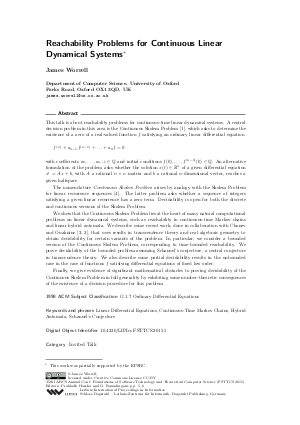Document# Reachability Problems for Continuous Linear Dynamical Systems (Invited Talk)

### Author James Worrell## File

LIPIcs.FSTTCS.2015.5.pdf
• Filesize: 274 kB
• 2 pages

## Cite As

James Worrell. Reachability Problems for Continuous Linear Dynamical Systems (Invited Talk). In 35th IARCS Annual Conference on Foundations of Software Technology and Theoretical Computer Science (FSTTCS 2015). Leibniz International Proceedings in Informatics (LIPIcs), Volume 45, pp. 5-6, Schloss Dagstuhl - Leibniz-Zentrum für Informatik (2015)
https://doi.org/10.4230/LIPIcs.FSTTCS.2015.5

## Abstract

This talk is about reachability problems for continuous-time linear dynamical systems. A central decision problem in the area is the Continuous Skolem Problem. In particular, this problem lies at the heart of several reachability questions in continuous-time Markov chains and linear hybrid automata. We describe some recent work, done in collaboration with Chonev and Ouaknine, that uses results in transcendence theory and real algebraic geometry to obtain decidability for certain variants of the problem. In particular, we consider a bounded version of the Continuous Skolem Problem, corresponding to time-bounded reachability. We prove decidability of the bounded problem assuming Schanuel's conjecture, a central conjecture in transcendence theory. We also describe some partial decidability results in the unbounded case in the case of functions satisfying differential equations of fixed low order. Finally, we give evidence of significant mathematical obstacles to proving decidability of the Continuous Skolem Problem in full generality by exhibiting some number-theoretic consequences of the existence of a decision procedure for this problem.
##### Keywords
• Linear Differential Equations
• Continuous-Time Markov Chains
• Hybrid Automata
• Schanuel's Conjecture

## Metrics

• Access Statistics
• Total Accesses (updated on a weekly basis)
0